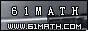Today is . WELCOME: huohai3983/44663  Home | Reg Login | All user | setHomePage | BookmarkHome | Math Test Online | Addition | Subtraction | Multiplication | Division | Time | Worksheet(JPG/PDF) | CAI | Flash Games |Recommendation articleArticle searchHome--maths--Third Grade--Adding Three Digit Numbers

Adding Three Digit Numbers

Adding Three Digit Numbers

Adding two three digit numbers (for example 529 + 733) involves several steps.

• Place one number above the other so that the hundreds, tens and ones places are lined up. Draw a line under the bottom number.
```
529
733

```
• Add the ones place digits (9 + 3 = 12). This number is larger than 10 so place a one above the tens place column and place the two below the line in the ones place column.
```
1
529
733
2
```
• Add the tens place digits (1 + 2 + 3 = 6) and place the answer below the line and in the tens place column.
```
529
733
62```
• Add the numbers in the hundreds place column (5 + 7 = 12) and place the 2 below the line and before the other number below the line. Place the 1 from the twelve above the thousands place column.
```
1
529
733
262```
• The thousands place column only has a 1 in it which should be placed below the line in the thousands place column.
```
529
733
1262```

#### Find the Sum of the two Numbers.

 +     =

2006-10-17 16:07:35
Viewed 2416 times CloseGreatmathsites

### Translate

 Thank you to online math channels' Mathematics Department for hosting these lessons. Copyright © 2006 - 2008 GZtrain Inc. All Rights Reserved